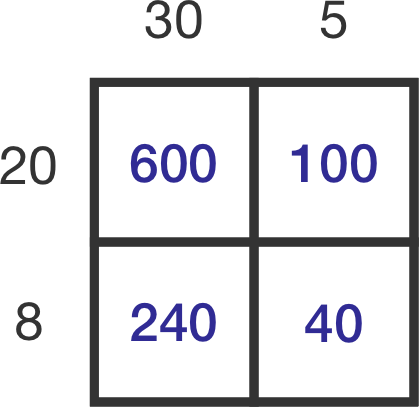Everyday Math

# Mental Shortcuts - Intermediate Warmup

Using the properties of decimals and the distributive rule, can you quickly find $15\%$ of 64?

Hint: $15 \% = 10\% + 5\%$.

Using the distributive rule, which of these are equivalent to $7 \times 64 + 7 \times 6?$

Using the perfect square identity, which of these is equivalent to $81^2 ?$

Hint: $(a+b)^2 = (a+b) \times ( a +b)$.

Using the difference of two squares identity, which of these is equivalent to $5.7 \times 6.3 ?$

Hint: $a^2 - b^2 = (a+b)(a-b)$.$35 \times 28 = \, ?$